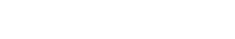# Thermoelectric Generator: Principle, Mechanism And Materials

Thermoelectric generators are solid state heat engines. They are made of two primary junctions, known as the p-type and n-type elements. The efficiency of the current generation is around 5-8%. Older devices used bimetallic junctions which lost power through heat.

We know that generators are used in the production of electricity, and the picture of a generator most of us have in mind is that they are huge machines with a magnetic field and a rotor that cuts through it with the help of mechanical forces to create electricity. However, what if I told you that electricity generation does not always require a machine with rotating parts? Let’s take a closer look at one such device, commonly known as the Thermoelectric Generator.The hot side of a thermoelectric generator (Photo Credit : Gerardtv/Wikimedia Commons)

## Principle

The thermoelectric effect is the direct conversion of heat into electricity. According to Joules Law, a current-carrying conductor produces heat proportional to the product of the resistance of the conductor and the square of the current passing through it. In the 1820s, Thomas J. Seebeck tested this law by interpreting it differently. He brought two dissimilar metals where the junctions at which the metals touch are of different temperatures. He noticed that a voltage developed between the junctions proportional to the difference in the heat. The current generated due to the difference in temperature at the junction of two different metals is known as the Seebeck Effect.The Seebeck Effect produces measurable amounts of voltage and current. The current density generated by a thermoelectric generator can be calculated with the following equation.The intensity of the electromotive field can be calculated using the Seebeck coefficient, which is inherently unique to each material being used, while delta T is the temperature gradient. Another effect that helps in describing the thermoelectric effect is the Peltier Effect.

The Peltier Effect helps in describing the heat dissipation or absorption at the connection of the conducting materials. Depending on the direction of the flow of current, heat is either dissipated or absorbed by that point in the material.

Also Read: What Are The Seebeck Effect And The Peltier Effect?

## Mechanism

The Seebeck Effect produces an electric current when dissimilar metals are exposed to a variance in temperature. Seebeck effect applications are the foundation of thermoelectric generators (TEGs) or Seebeck generators, which convert heat into energy. The voltage produced by TEGs or Seebeck generators is proportional to the temperature difference between the two metal junctions.

Thermoelectric generators are solid state heat engines made of two primary junctions, known as the p-type (high concentration of positive charge) and n-type (containing a high concentration of negative charge) elements. The p-type elements are doped in such a way to have a high number of positive charge or holes giving them a positive Seebeck coefficient. The n-type elements are doped to contain a high concentration of negative charge or electrons that give them a negative Seebeck coefficient.When an electrical connection occurs between the p-type element and the n-type element, for every hole that migrates to the n-type material, an electron from the n-type moves to the p-type material.

Also Read: What Are Semiconductors? And How Do They Work?

## Materials

Only very few elements to date have been identified as thermoelectric materials. Two important thermoelectric materials are Bismuth Telluride (Bi 2Te 3) at room temperature 9K (acting as the cold side) and Lead Telluride (PbTe), which is at 500K to 600K (acting as the hot side). These thermoelectric materials have a metric of measure that helps to evaluate the thermoelectric properties of the material; this measure is known as the figure of merit. The figure of merit for Bismuth Telluride (Bi 2Te 3) and Lead Telluride (PbTe) is one at the aforementioned temperatures. To be a viable source of credible power, the figure of merit must be 2 to 3.

There are numerous other factors that also need to be considered in the selection of a thermoelectric material. Ideally, a thermoelectric material must have a wide temperature gradient. If it does not have a wide temperature gradient, it will be susceptible to heat-induced stress, which might lead to the fracture of the material. The mechanical properties of the materials must be considered, and the coefficient of thermal expansion of the n-type and p-type materials must be matched reasonably well.The efficiency of the current generation in a thermoelectric generator is around 5-8%. Older devices were even less efficient, as they used bimetallic junctions, which lead to the severe loss of power through heat. More modern devices have a doped semiconductor material present within them, such as Lead Telluride (PbTe), Bismuth Telluride (Bi 2Te 3) and Calcium Manganese Oxide, or some combination of these materials.

Although thermoelectric power cannot compensate for mainstream power, it is helpful to a certain extent to use the latent energy lost as heat in a system as useful energy. While it may not be much, a little energy served over a long period of time can go a long way!

How well do you understand the article above!

Can you answer a few questions based on the article you just read?References (click to expand)